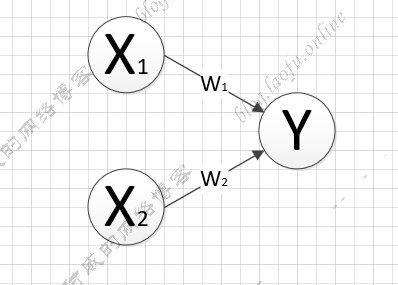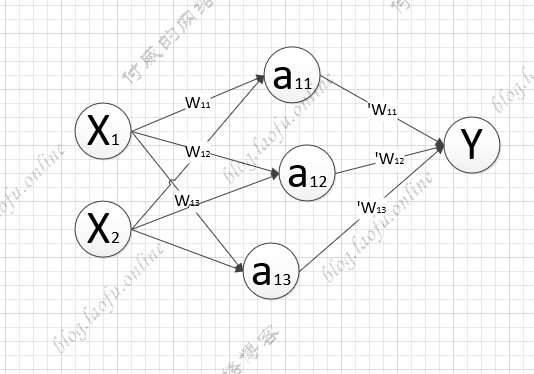# tensorflow的学习笔记--前向传播

### 输入参数w=tf.Variable(tf.random_normal([2,3],stddev=2,mean=0,seed=1))

truncated_normal：去掉过大偏离点的正态分布，如果生成的数据超过了平均值两个标准差，数据将重新生成。

random_uniform：平均分布

tf.zeros:生成全0数组，tf.zeros([3,2],int32) 生成[[0,0],[0,0],[0,0]] tf.ones:生成全1数组，tf.zeros([3,2],int32) 生成[[1,1],[1,1],[1,1]] tf.fill:全定值数组，tf.zeros([3,2],6) 生成[[6,6],[6,6],[6,6]] tf.constant:直接给值，tf.constant([3,2,1]) 生成[3,2,1]

### 神经网络的实现过程

1. 准备数据集，提取特征，作为输入喂给神经网络
2. 搭建NN结构，从输入到输出（先搭建计算图，再用会话执行）
(NN前向传播算法===»计算输出)
3. 大量特征数据喂给NN,迭代优化NN参数
（NN反向传播算法====»优化参数训练模型）

4. 使用训练好的模型预测和分类

### 前向传播输入参数X的权重矩阵$% $

隐藏层的矩阵$% $

隐藏层到输入结果的矩阵$'W=\left[ \begin{matrix} w_{11} \\ w_{12} \\ w_{13} \\ \end{matrix} \right]$

a=tf.matmaul(X，W)

Y=tf.matmaul(a,'W)

### 分析过程

1. 变量初始化，计算图节点，运算都需要sesion

2. 变量初始化：在session.run函数中，使用tf.global_variables_initializer()

 init_op=tf.global_variables_initializer()
sess.run(init_op)

3. 计算图节点运算:在sess.run函数中写入待运算的节点
sess.run(y)

4. 使用tf.placeholder占位，在sess.run函数中用feed_dict喂数据

喂一组数据：

x=tf.placeholder(tf.float32,shape=(1,2))
sess.run(y,feed_dict={x:[[0.5,0.6]]})


喂多组数据：

x=tf.placeholder(tf.float32,shape=(None,2))
sess.run(y,feed_dict={x:[[0.5,0.6],[0.5,0.6]]})


#coding:utf-8
# 两层神经网络
import tensorflow as tf

# 定义输入和参数
# 用placeholder实现喂数据
x=tf.placeholder(tf.float32,shape=(None,2))
w1=tf.Variable(tf.random_normal([2,3],stddev=1,seed=1))#正态分布随机数
w2=tf.Variable(tf.random_normal([3,1],stddev=1,seed=1))

#定义前向传播过程
a=tf.matmul(x,w1)
y=tf.matmul(a,w2)

# 计算结果
with tf.Session() as sess:
init_op=tf.global_variables_initializer()
sess.run(init_op)
print ("y in sj3 is:\n",sess.run(y,
feed_dict={
x:[[0.7,0.5],[0.2,0.3],[0.3,0.4],[0.4,0.5]]
}))


(本文完)